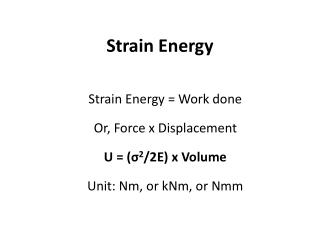DownloadDownload PresentationStrain Energy

# Strain Energy

Télécharger la présentation## Strain Energy

- - - - - - - - - - - - - - - - - - - - - - - - - - - E N D - - - - - - - - - - - - - - - - - - - - - - - - - - -
##### Presentation Transcript

1. Strain Energy Strain Energy = Work done Or, Force x Displacement U = (σ2/2E) x Volume Unit: Nm, or kNm, or Nmm

2. Strain Energy DUE TO • Elastic • Resilience • Proof Resilience • Modulus of Resilience • Loading • Gradual • Sudden • Impact • Shock • Effects • Shear • Bending • Torsion

3. Some definitions • ‘Elasticity’ can be defined as the ability of a body to store energy • ‘Resilience’ can be defined as the ability of a body to regain its original shape

4. Work Done = Work Stored Work Done = ½ P δl Work Stored = ½ σ A δl Strain Energy= ½ R δl P R δl

5. Strain Energy DUE TO Action • Gradual σ = P/A; u = (σ2/2E) V • Sudden σ = 2P/A; u = (σ2/4E) V • Impact σ = P/A + √[(P/A)2 + (2EPh)/(Al)]; u = ? • Shock u = (σ2/2E) V

6. Strain Energy DUE TO Effect • Shear u = (τ2/2G) x V • Bending u = 0∫l {(M2) dx / (2EI)} • Torsion u = (τ2/4G) x V  Solid Shaft u = (τ2/4G) x [(D2 + d2)/d2] x V  Hollow Shaft Draw Diagraames in Tutorial books.

7. Tutorials • Define • Strain Energy • Resilience • Impact Factor • Load Factor • State two differences between Proof Resilience (Up) and Modulus of Resilience (Um) • Derive to Work out ‘U’ i.e. strain energy due to Impact Loading • Draw neat diagrammes for Strain energy due to Shear, Bending and Torsion • Rethaliya Exercises. Page 463. Exercises 1, 2, 5 & 8